# Test: Mental Ability - 1

## 10 Questions MCQ Test Mathematics for Class 5: NCERT | Test: Mental Ability - 1

Description
Attempt Test: Mental Ability - 1 | 10 questions in 10 minutes | Mock test for Class 5 preparation | Free important questions MCQ to study Mathematics for Class 5: NCERT for Class 5 Exam | Download free PDF with solutions
QUESTION: 1

### Complete the series: 2, 5, 9, _________, 20, 27

Solution:

The pattern is + 3, + 4, + 5, + 6,.....
So, missing term = 9 + 5 = 14.

QUESTION: 2

### Three of the following are alike in certain way and so form a group. Which is the one that does not belong to that group.

Solution:

Except, wife, all others are people of one or more generation higher.

QUESTION: 3

### Modi is fourteenth from the right end in a row of 40 girls. What is his position from the left end?

Solution:

Ritu's rank from the right end = 14th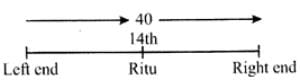So, Ritu's rank from the left end = (40-14) +1 = 27

QUESTION: 4

Modi is performing yoga with his head down and legs up. His face is towards the west. In which direction will his left hand be?

Solution:

Given that the face of Modi is towards the west.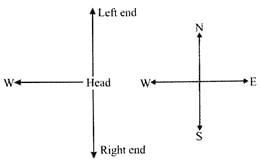Therefore, the left hand points towards north

QUESTION: 5

Choose the letter or group of letters which is different from others.

Solution:

Except Q, all other letters have even numbered positions in the English alphabet.

H - 8

T - 20

Z - 26

Q - 19

QUESTION: 6

Find the missing term in each of the following. 4,7,12,19,28,______

Solution: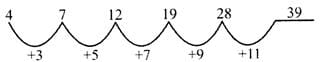QUESTION: 7

Three of the following are alike in certain way and so form a group. Which is the one that does not belong to that group?

Solution:

All the others are forms of vegetation.

QUESTION: 8

Choose the odd pair of words.

Solution:

All the other pair of words are rhyming words.

QUESTION: 9

P is 40 m south-west of Q. R is 40 m south-east of Q. Then R is in which direction of P?

Solution: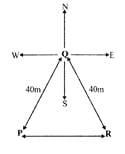QUESTION: 10

Rohani ranks seventh from the top and twenty sixth from the bottom in a class. How many students are there in the class?

Solution:

Rohani's rank from the top = 7th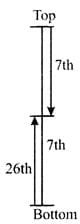Rohani's rank from the bottom = 26

So, Total number of students = (7+26)−1=33−1=32Use Code STAYHOME200 and get INR 200 additional OFF Use Coupon Code